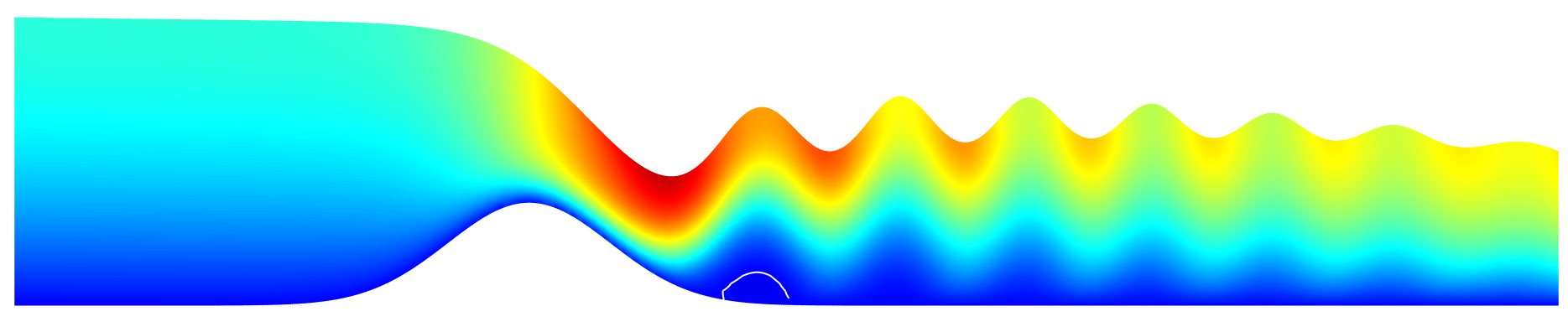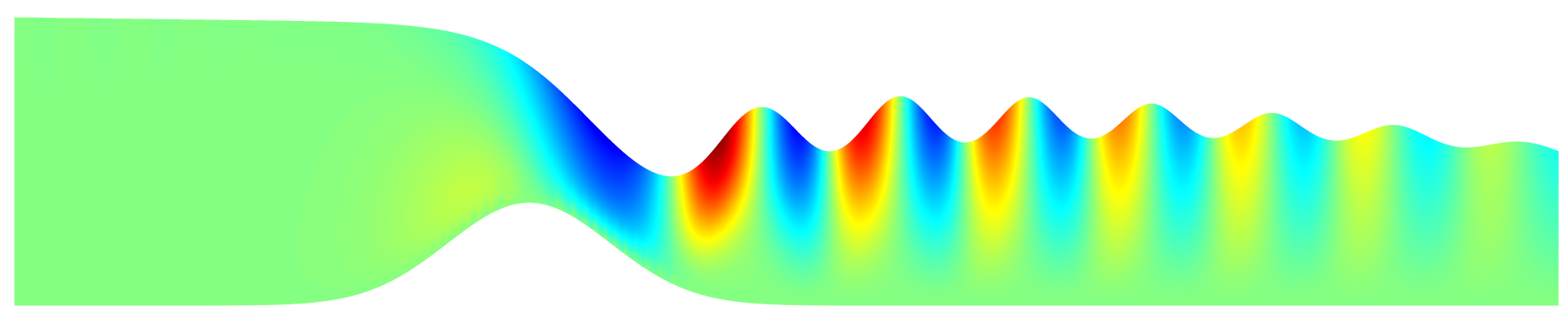# Transcritical flow over a bump

This is the Navier-Stokes/VOF version of this test case. A viscous fluid is injected to the left of a Gaussian bump and exits the domain with a fixed water depth. This creates a subcritical flow at inflow and outflow (i.e. the phase speed of gravity waves is larger than the fluid velocity) and a supercritical flow over the bump. In the absence of non-hydrostatic terms this would create a viscous hydraulic jump (see /src/test/gaussian.c). When non-hydrostatic terms are included (i.e. the full free-surface Navier-Stokes are solved), this leads to a steady wavetrain of dispersive waves.Horizontal velocity fieldVertical velocity field

See Popinet (2020) for a more detailed discussion.

#include "embed.h"
#include "navier-stokes/centered.h"
#include "two-phase.h"
#include "reduced.h"
#include "view.h"
#include "navier-stokes/perfs.h"

#define H0 0.6
#define QL 1.
#define BA 0.4
#define NU 1e-2

double hl = H0;

u.n[left] = dirichlet((t < 10. ? t/10. : 1.)*3./2.*QL/hl*(y < hl ? 1. - sq(y/hl - 1.) : 1.));
p[left] = neumann(0);
pf[left] = neumann(0);

u.n[right] = neumann(0.);
p[right]   = dirichlet(0.);
pf[right]  = dirichlet(0.);

u.n[embed] = dirichlet(0.);
u.t[embed] = dirichlet(0.);
u.t[bottom] = dirichlet(0.);

int main()
{
size (30);
N = 32;
rho1 = 0.001;
rho2 = 1.;
mu2 = NU;
mu1 = mu2*rho1/rho2/10.;
G.y = - 9.81;
Z.y = H0;
run();
}

event init (i = 0)
{
refine (y < 1.5 && level < 10);

fraction (f, y - H0);

solid (cs, fs, y - BA*exp(- sq(x - 10.)/5.) - 1e-3);
}

event update_hl (i++)
{
hl = 0.;
foreach_boundary (left, reduction(+:hl))
hl += Delta*f[];
hl = L0 - hl;
printf ("%g %g\n", t, hl);
}

event snapshot (i += 10; t <= 70) {
p.nodump = false;
dump();
}

event maxdt (t <= 10.; t += 0.05);

event profiles (t += 5)
{
fprintf (stderr, "# prof%g\n", t);
output_facets (f, stderr);
}

event pictures (t = end)
{
view (fov = 4.04484, tx = -0.498476, ty = -0.0923365, sy = 5,
bg = {1,1,1},
width = 1869, height = 390);
draw_vof ("cs", filled = -1, fc = {1,1,1});
draw_vof ("f", filled = 1, fc = {1,1,1});
squares ("u.x", min = -0.5, max = 4, linear = true);
isoline ("u.x", 0., lc = {1,1,1}, lw = 2);
save ("u.x.png");

draw_vof ("cs", filled = -1, fc = {1,1,1});
draw_vof ("f", filled = 1, fc = {1,1,1});
squares ("u.y", min = -0.8, max = 0.8, linear = true);
save ("u.y.png");
}